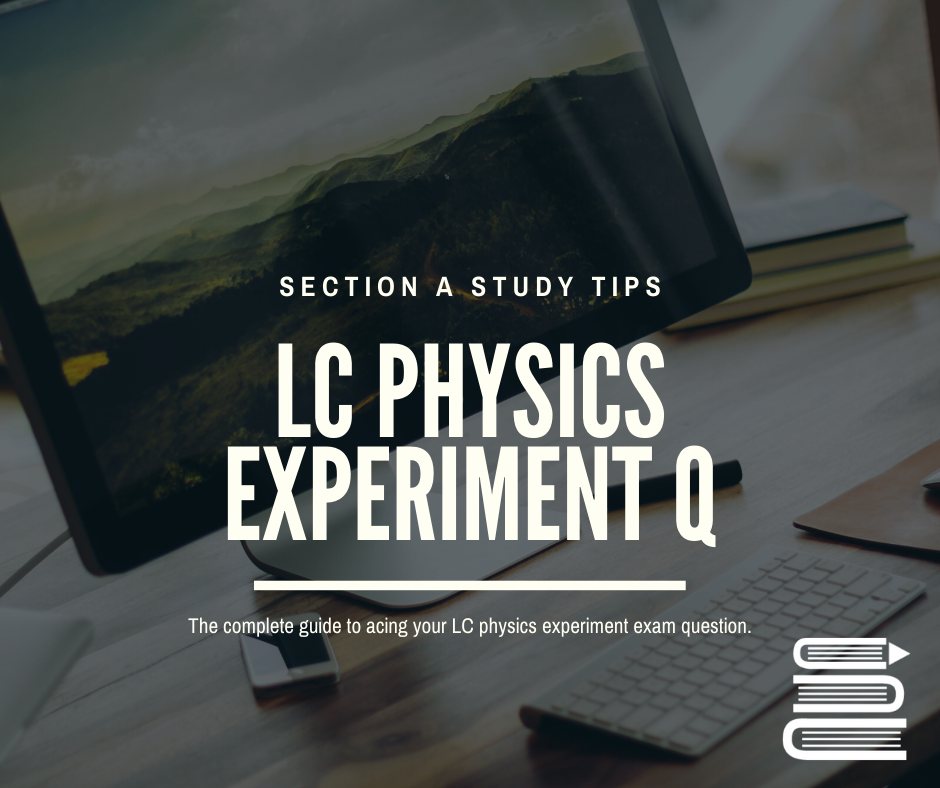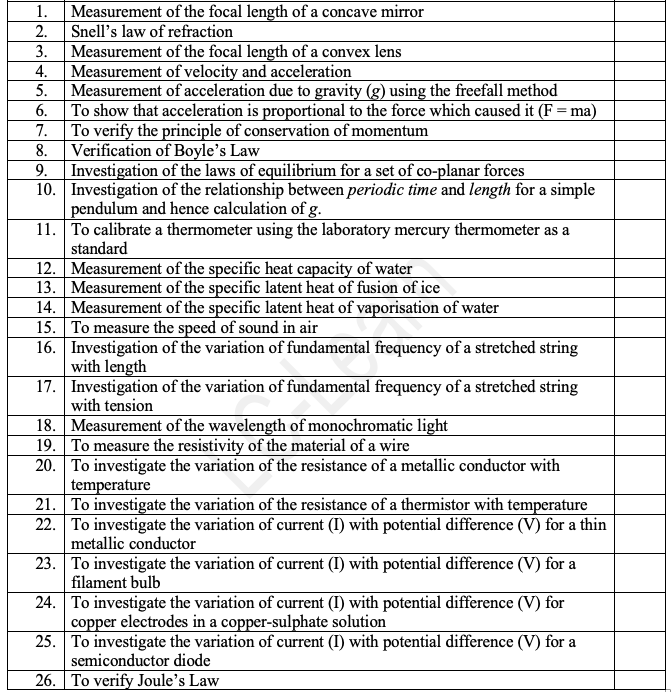# LC Physics Experiment Section Key Tips & Notes!Here is every mandatory experiment that could come up in this section A of the physics exam. Four experiments will come up and you must answer 3 questions. Mechanics and electricity almost always have an experiment question each year so we would recommend knowing all the experiments in those topics well.# Section A - Exam Technique

You will be given a set of results and will be asked to do some of the following:

1. Draw a labelled diagram.

2. Explain how the values were obtained.

3. To calculate some quantity (e.g. Specific Heat Capacity) or to verify a Law (e.g. Conservation of Momentum, Snell’s Law etc).

4. Some shorter questions on sources of error, precautions etc in relation to the performance of the experiment.

5. At least one of the questions will require a graph to be drawn. In such cases the slope of the graph will usually have to be calculated. The significance of the slope of the graph is determined by comparing it to a relevant formula (which links the two variables on the graph).

• Note: The data given will frequently have to be modified in some way (e.g. you may need to square one set of values or find the reciprocal etc) before the graph is drawn. This modification is determined by comparing it to the relevant formula which links the two variables.

When revising Section A make sure that you can do each of the following for every experiment:

• Draw a labelled diagram of the experimental set-up, including all essential apparatus. The first step in the procedures should then read “we set up the apparatus as shown in the diagram”.

• Describe how to obtain values for both sets of variables.

• Describe what needs to be adjusted to give a new set of data.

• Say what goes on the graph, and which variable goes on which axis.

• Know how to use the slope of the graph to obtain the desired answer (see below).

• List two or three precautions; if you are asked for two precautions, give three - if one is incorrect it will simply be ignored.

• List two or three sources of error.

### Miscellaneous Points:

• The graph question is usually well worth doing.

• Learn the following line off by heart as the most common source of error: “parallax error associated with using a metre stick to measure length / using a voltmeter to measure volts etc”.

• Make sure you understand the concept of percentage error; it’s the reason we try to ensure that what we’re measuring is as large as possible.

• There is a subtle difference between a precaution and a source of error – know the distinction.

• When asked for a precaution, do not suggest something which would result in giving no result, e.g. “Make sure the power-supply is turned on” (a precaution is something which could throw out the results rather than something which negates the whole experiment).

• To verify Joule’s Law does not involve a Joulemeter.

• To verify the Conservation of Momentum – the second trolley must be at rest.

• To verify the laws of equilibrium - the phrase ‘spring balance’ is not acceptable for ‘newton-metre’.

• To measure the Focal length of a Concave Mirror or a Convex Lens: Note that when given the data for various values of u and v, you must calculate a value for f in each case, and only then find an average. (As opposed to averaging the u’s and the v’s and then just using the formula once to calculate f).

### DRAWING THE GRAPH

• You must use graph paper and fill at least THREE QUARTERS OF THE PAGE.

• Use a scale which is easy to work with i.e. the major grid lines should correspond to natural divisions of the overall range.

• LABEL THE AXES with the quantity being plotted, including their units.

• Use a sharp pencil and mark each point with a dot, surrounded by a small circle (to indicate that the point is a data point as opposed to a smudge on the page.

• Generally all the points will not be in perfect line – this is okay and does not mean that you should cheat by putting them all on the line. Examiners will be looking to see if you can draw a best-fit line – you can usually make life easier for yourself by putting one end at the origin. The idea of the best-fit line is to imagine that there is a perfect relationship between the variables which should theoretically give a perfect straight line. Your job is to guess where this line would be based on the available points you have plotted.

• Buy a TRANSPARENT RULER to enable you to see the points underneath the ruler when drawing the best-fit line. Make that a LONG transparent ruler.

• DO NOT JOIN THE DOTS if a straight line graph is what is expected. Make sure that you know in advance which graphs will be curves.

• Note that examiners are obliged to check that each pint is correctly plotted, and you will lose marks if more than or two points are even slightly off.

• When calculating the slope choose two points that are far apart; usually the origin is a handy point to pick (but only if the line goes through it).

### WHAT GOES ON WHAT AXIS?

Option one:

• To show one variable is proportional to another, the convention is to put the independent variable on the x–axis, and the dependant variable on the y-axis, (from y = fn (x), meaning y is a function of x). The independent variable is the one which you control.

Option two:

• If the slope of the graph needs to be calculated then we use a difference approach, one which often contradicts option one, but which nevertheless must take precedence. In this case we compare a formula (the one which connects the two variables in question) to the basic equation for a line: y = mx.

There is usually a follow-up question like the following; “Draw a suitable graph on graph paper and explain how this verifies Snell’s Law”. There is a standard response to this;

• “The graph of Sin i against Sin r resulted in a straight line through the origin (allowing for experimental error), showing Sin i is directly proportional to Sin r, and therefore verifying Snell’s Law”.

If you are asked any questions to do with the information in the table, you are probably being asked to first find the slope of the graph, and use this to find the relevant information.

• And always, always, label your axes. *